Погода: 8 °C
 28.09 9...11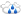29.09 10...13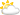Пробки: 4 баллаНГС.Форум /Форумы поддержки / Тестовый форум (Мусорка) /

# Жизнь струны (часть 6)

• большей дурости я не слышала, но это тааааак прикольно

Михаил Галустян. Это фсё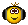И Наталья, Альбина, Галина
И Анна Кареновна из Сочи!

Еще не все погасли краски дня.
Еще не жаль огня,
И Бог хранит меня

•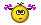что за п. 3?

By the way, how much is the fish? How much is the fish?
Here we go, here we go, here we go again! Yeah! Sunshine in the air!

•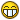феерично жеж

Еще не все погасли краски дня.
Еще не жаль огня,
И Бог хранит меня

• ага и продакт плейсмент мороженого чистая линия в серединеBy the way, how much is the fish? How much is the fish?
Here we go, here we go, here we go again! Yeah! Sunshine in the air!

• подозреваешь меня в работе на Чистую линию?Еще не все погасли краски дня.
Еще не жаль огня,
И Бог хранит меня

• Про любовь...
И они тоже были родоначальниками.

• Перекрёсток

• На стихи Боба Марли "I Shot The Sheriff "
Эрик Клэптон

• Очень странная песня

• Треш - Глеб Самойлоff & The Matrixx
ссылкой, ибо в текстах встречается п. 5Известно вам придурки, что давно
Про каждого снимается кино.

By the way, how much is the fish? How much is the fish?
Here we go, here we go, here we go again! Yeah! Sunshine in the air!

• WOODSTOCK 1969

• Jimi Hendrix

• Jethro Tull - давно не попадались!

• Выходят деды и не кривляясь, не выпрыгивая из штанов делают классную музыку.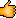• Все умерли. Остался один Палмер.

• Да вообще теперь здесь не интересно! когда всё под спойлерами!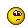• А можете ли вы спасти свою душу?

• Так было и нам казалось, что это что-то меняет в этом мире.

• Вымирают, как динозавры.

• - И меня не ослепит
Ложь в твоём краю.
Я иду искать мечту,
Чтобы жить в раю...

...Но не т рая на земле

• - Сочувствие не так уж много значит для меня.
Участие нечастый, верь, мой гость.
И если ищешь ты жилетку, куда поплакать,
Нет, это не ко мне.
Ведь у меня лишь моя музыка в цене

• Освободи меня, давай же, освободи меня,
Освободи меня, освободи меня от этих твоих чар.

• - Всё, что имел, я потерял.
Одиночество... вот мой финал.
Всё, что я думал, было моим...
Вернись ко мне, молю, давай повторим,
Вернись ко мне.

• Цыганка
Когда мне было семнадцать лет
Я влюбился в цыганскую королеву

• - Она пришла ко мне одним утром,
Одним одиноким воскресным утром.
Её длинные волосы плыли по ветру в середине зимы.
Я не знаю, как она меня нашла,
Ведь я шёл в темноте,
И всё вокруг меня лежало в разрухе
После боя, который я не cмог выиграть.

• Красивый старик!

Где мое "я", где мое "мы"?
Я сбился с пути, я опоздал на автобус.
Никто не знает, что будет дальше.
Куда делся завтрашний день
Откуда это берется
Я потерялся здесь, в Неверленде.
Я потерян, просто человек без плана.
Я потерян, потерян, потерян, я делаю все, что могу.
Но ветер судьбы суров.

Исправлено пользователем Сарра (26.11.22 22:12)

• Был красивый старик до 2020 года

• Показать скрытый текст
Скрыть текст

By the way, how much is the fish? How much is the fish?
Here we go, here we go, here we go again! Yeah! Sunshine in the air!

• By the way, how much is the fish? How much is the fish?
Here we go, here we go, here we go again! Yeah! Sunshine in the air!

• By the way, how much is the fish? How much is the fish?
Here we go, here we go, here we go again! Yeah! Sunshine in the air!

• В ответ на: - Сочувствие не так уж много значит для меня.
Есть еще одна СИМПАТИчная песня с таким же названием у другой группы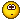Тулмбтнг!

• Ну, мелодия слишком известна, чтобы её перепутать с хиповской вещугой (которая не менее известна). Её очень любила советская пропаганда. Её совали фоном во все антиимпериалистические документальные фильмы и хронику. Ну и она воспринималась скорее как попсовая мелодия. С роком не ассоциировалась.
Показать скрытый текст
Сочувствие – вот все, что нужно нам
Сочувствие – и это все
Сочувствие – вот все, что нужно нам
слишком мало любви, чтобы жить
не хватает любви, чтобы жить

Полмира ненавидит остальных
И у одних все в жизни есть
А у других лишь голод и печаль
слишком мало любви, чтобы жить
не хватает любви, чтобы жить
Скрыть текст

•Собчак:

День рожденья Бориса Борисовича Гребенщикова — повод напомнить, от какого крутого варианта обложки альбома «Радио Африка» когда-то он отказался. Сами понимаете - от которого отказался. Для советского 1983-го и российского 2022-го радикально. Как говорится, рок-н-ролл мёртв, а я ещё нет.

By the way, how much is the fish? How much is the fish?
Here we go, here we go, here we go again! Yeah! Sunshine in the air!

•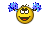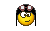By the way, how much is the fish? How much is the fish?
Here we go, here we go, here we go again! Yeah! Sunshine in the air!

•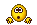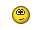By the way, how much is the fish? How much is the fish?
Here we go, here we go, here we go again! Yeah! Sunshine in the air!

• By the way, how much is the fish? How much is the fish?
Here we go, here we go, here we go again! Yeah! Sunshine in the air!

• By the way, how much is the fish? How much is the fish?
Here we go, here we go, here we go again! Yeah! Sunshine in the air!

•By the way, how much is the fish? How much is the fish?
Here we go, here we go, here we go again! Yeah! Sunshine in the air!

• By the way, how much is the fish? How much is the fish?
Here we go, here we go, here we go again! Yeah! Sunshine in the air!

• By the way, how much is the fish? How much is the fish?
Here we go, here we go, here we go again! Yeah! Sunshine in the air!

•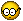By the way, how much is the fish? How much is the fish?
Here we go, here we go, here we go again! Yeah! Sunshine in the air!

• By the way, how much is the fish? How much is the fish?
Here we go, here we go, here we go again! Yeah! Sunshine in the air!

•By the way, how much is the fish? How much is the fish?
Here we go, here we go, here we go again! Yeah! Sunshine in the air!

• Вышли два старпёра. Они не могли скакать. Но! Они могли делать такую музыку, от которой стадионы писались кипятком! И никто до них до сих пор не дотянулся ни разу.

Исправлено пользователем Сарра (27.11.22 22:36)

• Борис Гребенщиков в свой день рождения поставил слушателям «Аэростата» десять своих любимых, но не звучавших за все 17,5 лет в передаче композиций

«Сегодня мой день рождения, и я хочу разделить с вами те озарения (или помрачнения, можете называть как хотите), которые удивляют, потрясают и развлекают меня в последнее время. И сопроводить их музыкой, которую я люблю всем сердцем, но почему-то не удосужился вам поставить за эти 17 с половиной лет», - заявил музыкант.

Вот что поставил БГ:

- Beatles – I've Just Seen A Face
- Jimi Hendrix – Remember
- George Harrison – Drilling A Home
- Jethro Tull – Back-Door Angels
- Rolling Stones – Saint Of Me
- George Harrison – Soft Touch
- Robert Palmer – History
- Pugwash – Omega Man
- Donovan – Guinevere
- New Vaudeville Band – Green Street Green

Ну а редакция "Фонтанки" присоединяется к поздравлениям!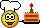@fontankaspb

By the way, how much is the fish? How much is the fish?
Here we go, here we go, here we go again! Yeah! Sunshine in the air!

• Гимн выходныхOne shot, bring me more
Two shot, let 'em roll
Three, four, five, six, seven, eight, nine...

... I'm okay
I'm not alcoholic

Девочка серии не-расти-после-меня-трава...

• Циничный подонок какой-то.

• Сладких снов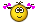By the way, how much is the fish? How much is the fish?
Here we go, here we go, here we go again! Yeah! Sunshine in the air!

Записей на странице:

Модераторы: